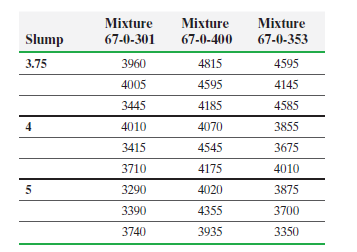×
Get Full Access to Statistics: Informed Decisions Using Data - 5 Edition - Chapter 13.4 - Problem 19
Get Full Access to Statistics: Informed Decisions Using Data - 5 Edition - Chapter 13.4 - Problem 19

×

# ?Concrete Strength An engineer wants to know if the mean strengths of three different concrete mix designs differ significantly. He also suspects thatISBN: 9780134133539 240

## Solution for problem 19 Chapter 13.4

Statistics: Informed Decisions Using Data | 5th Edition

• Textbook Solutions
• 2901 Step-by-step solutions solved by professors and subject experts
• Get 24/7 help from StudySoup virtual teaching assistantsStatistics: Informed Decisions Using Data | 5th Edition

4 5 1 296 Reviews
24
2
Problem 19

Concrete Strength An engineer wants to know if the mean strengths of three different concrete mix designs differ significantly. He also suspects that slump may be a predictor of concrete strength. Slump is a measure of the uniformity of the concrete, with a higher slump indicating a less uniform mixture. The following data represent the 28-day strength (in pounds per square inch) of three different mixtures with three different slumps.(a) Normal probability plots indicate that it is reasonable to believe that the data come from populations that are normally distributed. Verify the requirement of equal population variances

(b) Determine whether there is significant interaction between mixture type and slump.

(c) If there is no significant interaction, determine whether there is significant difference in the means for the three types of mixture. If there is no significant interaction, determine whether there is significant difference in the means for the slumps.

(d) Draw an interaction plot of the data to support the results of parts (b) and (c).

(e) The residuals are normally distributed. Verify this.

(f) If there is significant difference in the means for the three mixture types, use Tukey’s test to determine which pairwise means differ using a familywise error rate of a = 0.05. If there is significant difference in the means for the slumps, use Tukey’s test to determine which pairwise means differ using a familywise error rate of a = 0.05.

Step-by-Step Solution:

Step 1 of 5) Concrete Strength An engineer wants to know if the mean strengths of three different concrete mix designs differ significantly. He also suspects that slump may be a predictor of concrete strength. Slump is a measure of the uniformity of the concrete, with a higher slump indicating a less uniform mixture. The following data represent the 28-day strength (in pounds per square inch) of three different mixtures with three different slumps. (a) Normal probability plots indicate that it is reasonable to believe that the data come from populations that are normally distributed. Verify the requirement of equal population variances (b) Determine whether there is significant interaction between mixture type and slump. (c) If there is no significant interaction, determine whether there is significant difference in the means for the three types of mixture. If there is no significant interaction, determine whether there is significant difference in the means for the slumps. (d) Draw an interaction plot of the data to support the results of parts (b) and (c). (e) The residuals are normally distributed. Verify this. (f) If there is significant difference in the means for the three mixture types, use Tukey’s test to determine which pairwise means differ using a familywise error rate of a = 0.05.

Step 2 of 2

## Discover and learn what students are asking

Chemistry: The Central Science : Introduction:Matter, Energy, and Measurement
?Which of the following diagrams represents a chemical change? [Section 1.3]

Statistics: Informed Decisions Using Data : Inference about the Difference between Two Medians: Dependent Samples
?In Problems 3–10, use the Wilcoxon matched-pairs signedranks test to test the given hypotheses at the a = 0.05 level of significance. The dependent sa

Unlock Textbook Solution